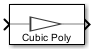# Memoryless Nonlinearity

Apply memoryless nonlinearity to complex baseband signal

• Library:
• Communications Toolbox / RF Impairments

•## Description

The Memoryless Nonlinearity block applies memoryless nonlinear impairments to a complex baseband signal. Use this block to model memoryless nonlinear impairments caused by signal amplification in the radio frequency (RF) transmitter or receiver. For more information, see Memoryless Nonlinear Impairments.

Note

All values of power assume a nominal impedance of 1 ohm.

## Ports

### Input

expand all

Input RF baseband signal, specified as a scalar or column vector. Values in this input must be complex.

Data Types: `double`
Complex Number Support: Yes

### Output

expand all

Output RF baseband signal, returned as a scalar or column vector. The output is of the same data type as the input.

## Parameters

expand all

Nonlinearity modeling method, specified as `Cubic polynomial`, `Hyperbolic tangent`, `Saleh model`, `Ghorbani model`, `Rapp model`, or `Lookup table`. For more information, see Memoryless Nonlinear Impairments.

Linear gain in decibels, specified as a scalar. This parameter scales the power gain of the output signal.

Tunable: Yes

#### Dependencies

To enable this parameter, set the Method to `Cubic polynomial`, ```Hyperbolic tangent```, or `Rapp model`.

Data Types: `double`

Third-order input intercept point in dBm, specified as a scalar.

Tunable: Yes

#### Dependencies

To enable this parameter, set the Method to `Cubic polynomial` or ```Hyperbolic tangent```.

Data Types: `double`

AM/PM conversion factor in degrees per decibel, specified as a scalar. For more information, see Cubic Polynomial and Hyperbolic Tangent Model Methods.

Tunable: Yes

#### Dependencies

To enable this parameter, set the Method to `Cubic polynomial` or ```Hyperbolic tangent```.

Data Types: `double`

Input power lower limit in dBm, specified as a scalar less than the Upper input power limit for AM/PM conversion (dBm) parameter value. The AM/PM conversion scales linearly for input power values in the range [Lower input power limit for AM/PM conversion (dBm), Upper input power limit for AM/PM conversion (dBm)]. If the input signal power is below the input power lower limit, the phase shift resulting from AM/PM conversion is zero. For more information, see Cubic Polynomial and Hyperbolic Tangent Model Methods.

Tunable: Yes

#### Dependencies

To enable this parameter, set the Method to `Cubic polynomial` or `Hyperbolic tangent`.

Data Types: `double`

Input power upper limit in dBm, specified as a scalar greater than the Lower input power limit for AM/PM conversion (dBm) parameter value. The AM/PM conversion scales linearly for input power values in the range [Lower input power limit for AM/PM conversion (dBm), Upper input power limit for AM/PM conversion (dBm)]. If the input signal power is below the input power lower limit, the phase shift resulting from AM/PM conversion is zero. For more information, see Cubic Polynomial and Hyperbolic Tangent Model Methods.

Tunable: Yes

#### Dependencies

To enable this parameter, set the Method to `Cubic polynomial` or `Hyperbolic tangent`.

Data Types: `double`

Input signal scaling factor in decibels, specified as a scalar. This parameter scales the power gain of the input signal.

Tunable: Yes

#### Dependencies

To enable this parameter, set the Method to `Saleh model` or ```Ghorbani model```.

Data Types: `double`

AM/AM parameters for Saleh model, used to compute the amplitude gain for an input signal, specified as a two-element vector. For more information, see Saleh Model Method.

Tunable: Yes

#### Dependencies

To enable this parameter, set the Method to `Saleh model`.

Data Types: `double`

AM/PM parameters for Saleh model, used to compute the phase change for an input signal, specified as a two-element vector. For more information, see Saleh Model Method.

Tunable: Yes

#### Dependencies

To enable this parameter, set the Method to `Saleh model`.

AM/AM parameters for Ghorbani model, used to compute the amplitude gain for an input signal, specified as a four-element vector. For more information, see Ghorbani Model Method.

Tunable: Yes

#### Dependencies

To enable this parameter, set the Method to `Ghorbani model`.

Data Types: `double`

AM/PM parameters for Ghorbani model, used to compute the phase change for an input signal, specified as a four-element vector. For more information, see Ghorbani Model Method.

Tunable: Yes

#### Dependencies

To enable this parameter, set the Method to `Ghorbani model`.

Data Types: `double`

Output signal scaling factor in decibels, specified as a scalar. This parameter scales the power gain of the output signal.

Tunable: Yes

#### Dependencies

To enable this parameter, set the Method to `Saleh model` or ```Ghorbani model```.

Data Types: `double`

Smoothness factor, specified as a scalar. For more information, see Rapp Model Method.

Tunable: Yes

#### Dependencies

To enable this parameter, set the Method to `Rapp model`.

Data Types: `double`

Output saturation level, specified as a scalar. For more information, see Rapp Model Method.

Tunable: Yes

#### Dependencies

To enable this parameter, set the Method to `Rapp model`.

Data Types: `double`

## Block Characteristics

 Data Types `double` | `single` Multidimensional Signals `no` Variable-Size Signals `no`